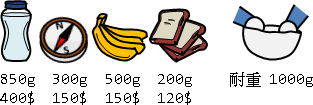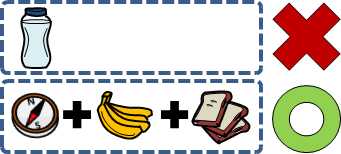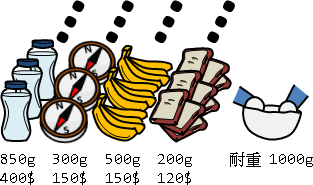Knapsack Problem

Knapsack ProblemFractional Knapsack Problem

Fractional Knapsack Problem

Fractional是「分數」的意思。一個物品可以切下一部分、只取幾分之幾放進背包。

0/1 Knapsack Problem

0/1 Knapsack Problem

「0/1」的意思是：每種物品只會放進背包零個或一個。一個物品要嘛整個不放進背包、要嘛整個放進背包。物品無法切割。0/1背包問題的關鍵點，在於如何有效利用背包的剩餘重量，找出最好的物品組合方式。

0/1背包問題是經典的NP-complete問題，無法快速求得精確解，只能折衷求得近似解。然而，當數值範圍不大時，得以用動態規劃快速求得精確解。

```讓背包裡面的物品總價值最大

```

UVa 431 624 990 10130 10819 10980```c(n, w) = max( c(n-1, w), c(n-1, w-weight[n]) + cost[n] )
^^^^^^^^^  ^^^^^^^^^^^^^^^^^^^^^^^^^^^^^
不放 -> 0            有放 -> 1

n：第0個到第n個物品要放進背包內。
w：背包耐重限制。
c(n, w)：只有第0個到第n個物品，耐重限制為w，此時的背包問題答案。
weight[n]：第n個物品的重量。
cost[n]：第n個物品的價值。
```

```c(n, w) =
{ -INF                                   , if w < 0
{ -INF                                   , if n < 0
{ 0                                      , if n = 0 and w >= 0
{ max( c(n-1, w),                        , if n > 0 and w >= 0
{      c(n-1, w-weight[n]) + cost[n] )
```

```c(n, w) =
{ 0                                      , if n = 0
{ c(n-1, w)                              , if n > 0
{                                          and w-weight[n] < 0
{ max( c(n-1, w),                        , if n > 0
{      c(n-1, w-weight[n]) + cost[n] )     and w-weight[n] >= 0
```

Backtracking，窮舉所有組合方式。時間複雜度O(NA)，A是組合方式數量。

```c(n, w, m) = max( c(n-1, w-weight[n], m-1) + cost[n] , c(n-1, w, m) )

m：放進背包的物品個數。
```

```c(n, w) =
{ -INF                                   , if n < 0
{ -INF                                   , if w < 0
{ -INF                                   , if n = 0 and w != 0
{                                                   ^^^^^^^^^^
{ 0                                      , if n = 0 and w = 0
{                                                   ^^^^^^^^^
{ max( c(n-1, w),                        , if n > 0
{      c(n-1, w-weight[n]) + cost[n] )

n：第0個到第n個物品要放進背包內。
w：放進背包內的物品總重量！
c(n, w)：只有第0個到第n個物品，背包內的物品總重量為w，此時的背包問題答案！
weight[n]：第n個物品的重量。
cost[n]：第n個物品的價值。
```

```w(n, c) =
{ +INF                                   , if n < 0 or  c < 0
{ 0                                      , if n = 0 and c = 0
{ min( w(n-1, c),                        , if n > 0
{      w(n-1, c-cost[n]) + weight[n] )

n：第0個到第n個物品要放進背包內。
w：放進背包內的物品總重量！
c(n, w)：只有第0個到第n個物品，背包內的物品總重量為w，此時的背包問題答案！
weight[n]：第n個物品的重量。
cost[n]：第n個物品的價值。
```

```c(n, w) = max(
0 ,                                 都不放
c(0, w-weight) + cost ,       最後是放第0個物品
c(1, w-weight) + cost ,       最後是放第1個物品
... ,                               ...
c(n-1, w-weight[n-1]) + cost[n-1]   最後是放第n-1個物品
)

n：第0個到第n個物品要放進背包內。
w：背包負重上限。
c(n, w)：只有第0個到第n個物品，負重限制為w，此時的背包問題答案。
weight[n]：第n個物品的重量。
cost[n]：第n個物品的價值。
```

【待補文字】

```polynomial-time approximation scheme
http://www.cse.cuhk.edu.hk/~chi/csc5160-2008/notes/L17-PTAS.pdf

cost 超級大  沒辦法用DP解的時候

```

UVa 10715

```一、建立輔助表格，處理附加的條件。

```

Unbounded Knapsack ProblemUVa 10465

```c(n, w) = max(
c(n-1, w - weight[n] ⋅ 0) + cost[n]     ,   用去零個第n種物品
c(n-1, w - weight[n] ⋅ 1) + cost[n] ⋅ 1 ,   用去一個第n種物品
c(n-1, w - weight[n] ⋅ 2) + cost[n] ⋅ 2 ,   用去兩個第n種物品
... ,                                       ...
)

n：第0種到第n種物品要放進背包內。
w：背包耐重限制。
c(n, w)：只有第0種到第n種物品，耐重限制為w，此時的背包問題答案。
weight[n]：第n種物品的重量。
cost[n]：第n種物品的價值。
```

```c(n, w) = max( c(n-1, w), c(n, w-weight[n]) + cost[n] )
^^
只有這裡不同，因為物品無限量供應。
```

UVa 10898

Bounded Knapsack Problem```c(n, w) = max(
c(n-1, w - weight[n] ⋅ 0) + cost[n] ⋅ 0 ,   用去零個第n種物品
c(n-1, w - weight[n] ⋅ 1) + cost[n] ⋅ 1 ,   用去一個第n種物品
... ,                                       ...
c(n-1, w - weight[n] ⋅ number[n])           用去number[n]個第n種物品
+ cost[n] ⋅ number[n]
)

n：第0種到第n種物品要放進背包內。
w：背包耐重限制。
c(n, w)：只有第0種到第n種物品，耐重限制為w，此時的背包問題答案。
weight[n]：第n種物品的重量。
cost[n]：第n種物品的價值。
number[n]：第n種物品的數量。
```

UVa 10086

Scaling Method。同種類的M個物品，實施二進位分解，重捆成1、2、4、8、……、2ᵏ、M - 2ᵏ個物品，一共⌈logM⌉捆。這些捆的0/1組合，可以湊出各種數量的物品。

http://www.cnblogs.com/GXZC/archive/2013/01/08/2851153.html

http://morris821028.github.io/2016/12/18/jg-20008/

Money Changing Problem

```能否湊得某個價位（Money Changing Problem）

```Money Changing Problem其實就是Unbounded Knapsack Problem的變形：物品變成錢幣，物品重量變成面額，物品價值變成「湊得到、湊不到」兩種情況，累計價值的方式從加法運算變成OR運算。

```c(n, m) = c(n-1, m) or c(n, m-price[n])
^^^^^^^^^    ^^^^^^^^^^^^^^^^
不用這種錢幣    用去一個錢幣

n：用第0種到第n種錢幣來湊得價位。
m：欲湊得的價位值。
c(n, m)：用第0種到第n種錢幣湊得價位m的湊法數目。
price[n]：第n種錢幣的面額大小。
```

UVa 10306 10898 10261

```c(n, m) = c(n-1, m) + c(n, m-price[n])
^^^^^^^^^   ^^^^^^^^^^^^^^^^
不用這種錢幣    用去一個錢幣

c(n, m)：用第0種到第n種錢幣湊得價位m的湊法數目。
```

UVa 147 357 674 10313 430

```c(n, m) = min( c(n-1, m) , c(n, m-price[n]) + 1 )
^^^^^^^^^   ^^^^^^^^^^^^^^^^^^^^
保持一樣        用去一個錢幣

c(n, m)：用第0種到第n種錢幣湊得價位m，最少所需要的錢幣數量。
```

UVa 242 10032 10690 10930

UVa 233 711

Sebastian Böcker, Zsuzsanna Lipták. A Fast and Simple Algorithm for the Money Changing Problem. Algorithmica, 2007, 48, 413-432.

【待補文字】

【待補文字】

Change-Making Problem

UVa 166

```一、各種價錢都能找，不會有找不出來的情況。

```

```【問題描述】

【性質甲】正確湊法有最佳子結構（包含關係）

【性質乙】貪心湊法是嚴格遞增函數（字典順序關係）

（以高項次代表高位數。）

【性質丙】b(M) 與 g(M) 剛好不相交
b(M) AND g(M) EQUAL (0, 0, 0, ...., 0)
b(M) 和 g(M) 對應的項不會同時有值，因為拿掉這些錢幣，M 就可以更小，矛盾。

【證明開始】

bs 與 bt 都大於零，bs 的左邊都是零，bt 的右邊都是零。

【證明步驟一】M 之下限

【證明步驟二】M 之上限

（也可以推得 (M - ct) < ct+1 ，不過他比較寬鬆，用不到。）

【證明步驟三】觀察 (ct+1 - 1) 的湊法。使用夾擠。
M > (ct+1 - 1) >= (M - cs)　【步驟一二】
g(M) > g(ct+1 -1) >= g(M - cs)，如果 g(M) 是對的湊法。【性質乙】

b(M) > g(ct+1 - 1) >= b(M - cs)

【步驟三補充】證明 b(M) > g(ct+1 - 1 - ct) 在第 t 項加一

【證明步驟四】
b(M) 與 b(M - cs) 幾乎一樣。【性質甲】【M的前提】

b(M)        = ( ..., 0 , gs    , ... , gt, 0 , ... )
>  g(ct+1 - 1) = ( ???, ? , gs - 1, ... , gt, 0 , ... )
>= b(M - cs)   = ( ..., 0 , gs - 1, ... , gt, 0 , ... )

g(ct+1 - 1) 被夾在中間，根據字典順序關係【性質乙】，從第 s 項以上都是確定值。

【演算法】
M = +oo
for i = 1 to n
算 g(ci+1 - 1) = b(ci+1 - 1)。  // 一定是對的湊法。
for j = 1 to i                  // 窮舉第 s 項的位置。
算 b(X)，讓 g(ci+1 - 1) 第 j-1 項以下都變成零，讓第 j 項加一，即得。
算 X。
算 g(X)。
if g(X) 與 b(X) 的錢幣用量相同（項次不同沒關係）
X可以湊出正確值，不是我們要的。不做任何事。
else
更新 M，M = min(M, X)。
return M
```

Partition Problem

```一個數字集合，挑幾個數字，總和恰為零（Subset Sum Problem）

N個不同重量物品，M個不同耐重箱子，用最少箱子裝所有物品（Bin Packing Problem）
```

Banzhaf Power Index

Banzhaf Power Index的計算方式是這樣的：一個派系X的Banzhaf Power Index = 派系X影響決策的情況數目 ÷ (派系1影響決策的情況數目 + ... + 派系N影響決策的情況數目)。所有派系的Banzhaf Power Index的總和會是1。

```1.
A派系9票、B派系9票、C派系7票、D派系3票、E派系1票、F派系1票。

2.

AB AC ABC ABD ABE ABF ACD ACE ACF
ABDE ABDF ABEF ACDE ACDF ACEF ADEF

3.

| votes | power |  BPI
--+-------+-------+-------
A |   9   |  16   | 16/48
B |   9   |  16   | 16/48
C |   7   |  16   | 16/48
D |   3   |   0   |   0
E |   1   |   0   |   0
F |   1   |   0   |   0
--+-------+-------+--------
|  30   |  48   |  1.0
```

```甲、移除此派系。

```

UVa 430 435